# Object detection and detection heads

Last updated on：8 months ago

Object detection has a significant relationship with video analysis and image understanding. Region selection is an essential part of object detection. Usually, we call it a (detection) head to distinguish it from classification and segmentation (head).

# Introduction

Object detection is an important computer vision task that deals with detecting instances of visual objects of a certain class (such as humans, animals, or cars) in digital images.
Object detection is a task that precisely estimates the concepts and locations of objects in each image.
Object detection provides information for the semantic understanding of images and videos and is related to many applications, including image classification, human behaviour analysis, face recognition, and autonomous driving.
Object detection answers the question, “what objects are where”.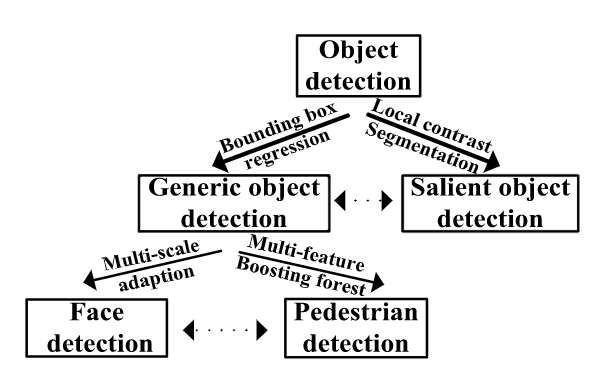## Generic object detection

Generic object detection aims to locate and classify existing objects in any one image and label them with rectangular BBs to show their confidence.
Pipeline:

• (First type) Generating region proposals first and then classifying each proposal into different object categories.
• (Second type) Adopting a unified framework to achieve final results (categories and locations) directly.

## Detection stages

• Informative region selection.
• Feature extraction.
• Classification.

# Region proposal-based framework

The region proposal-based framework, a two-stage detector, matches the attentional mechanism of the human brain to some extent, which gives a coarse scan of the whole scenario first and then focuses on regions of interest (ROI).
Flowchart of R-CNN, consisting of three stages: 1) extracts BU region proposals, 2) computes features for each proposal using a CNN, and 3) classifies each region with class-specific linear SVMs.
Region proposal-based framework for detection includes R-CNN, Spatial pyramid pooling (SPP)-net, Fast R-CNN, Faster R-CNN, region-based fully convolutional network (R-FCN), feature pyramid networks (FPN), and Mask R-CNN.

## SPP-net

Spatial pyramid pooling (SPP) is used to eliminate artificial fixed-size (e.g., $224 \times 224$) input images reducing the recognition accuracy for the images or sub-images of an arbitrary size/scale.
SPP-net computes the feature maps from the entire image only once, and then pool features in arbitrary regions (sub-images) to generate fixed-length representations for training the detectors.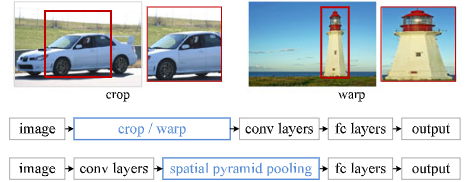Network structure with spatial pyramid pooling layer.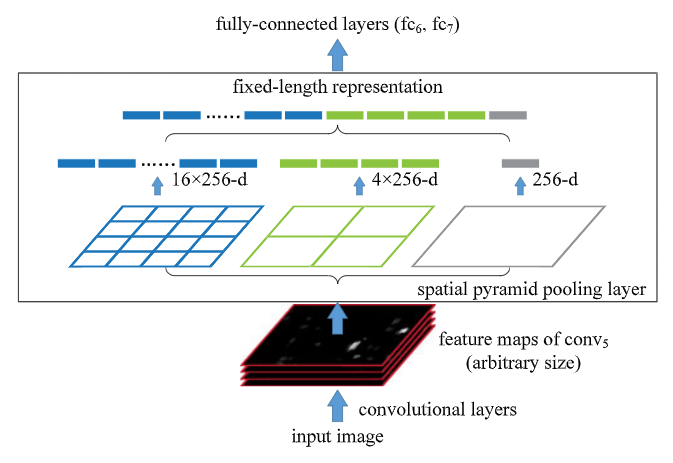Codes:

import math

def spatial_pyramid_pool(self,previous_conv, num_sample, previous_conv_size, out_pool_size):
'''
previous_conv: a tensor vector of the previous convolution layer
num_sample: an int number of images in the batch
previous_conv_size: an int vector [height, width] of the matrix features a size ofthe  previous convolution layer
out_pool_size: an int vector of expected output size of max pooling layer

returns: a tensor vector with shape [1 x n] is the concentration of multi-level pooling
'''
# print(previous_conv.size())
for i in range(len(out_pool_size)):
# print(previous_conv_size)
h_wid = int(math.ceil(previous_conv_size / out_pool_size[i]))
w_wid = int(math.ceil(previous_conv_size / out_pool_size[i]))
h_pad = (h_wid * out_pool_size[i] - previous_conv_size + 1)/2
w_pad = (w_wid * out_pool_size[i] - previous_conv_size + 1)/2
x = maxpool(previous_conv)
if(i == 0):
spp = x.view(num_sample, -1)
# print("spp size:",spp.size())
else:
# print("size:",spp.size())
spp = torch.cat((spp,x.view(num_sample,-1)), 1)
return spp

## R-CNN

Regions with CNN features (R-CNN) consists of three modules:

• Generate category-independent region proposals. These proposals define the set of candidate detections available to our detector.
• A large convolutional neural network that extracts a fixed-length feature vector from each region.
• A set of class specific linear SVMs.
While R-CNN is agnostic to the particular region proposal method, we use selective search to enable a controlled comparison with prior detection work.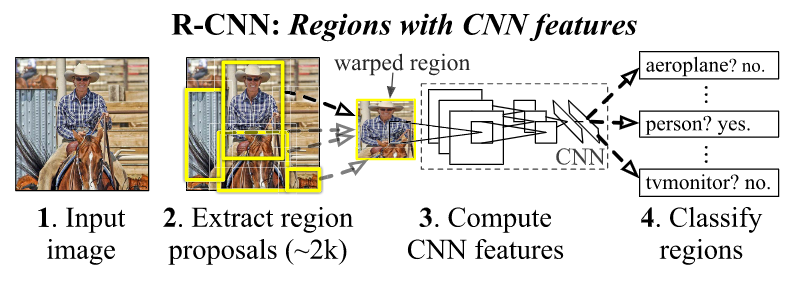## Fast R-CNN

Fast region-based convolutional network method (Fast R-CNN) training in single-stage, using a multi-task loss, which can update all layers.
An input image and multiple regions of interest (ROIs) are input into a fully convolutional network. Each ROI is pooled into a fixed-size feature map and then mapped to a feature vector by fully connected layers (FCs). Fast R-CNN has two output vectors per ROI: softmax probabilities and per-class bounding-box regression offsets.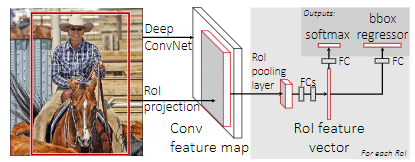Codes:

class _ProposalTargetLayer(nn.Module):
def forward(self, all_rois, gt_boxes, num_boxes):
self.BBOX_NORMALIZE_MEANS = self.BBOX_NORMALIZE_MEANS.type_as(gt_boxes)
self.BBOX_NORMALIZE_STDS = self.BBOX_NORMALIZE_STDS.type_as(gt_boxes)
self.BBOX_INSIDE_WEIGHTS = self.BBOX_INSIDE_WEIGHTS.type_as(gt_boxes)

gt_boxes_append = gt_boxes.new(gt_boxes.size()).zero_()
gt_boxes_append[:,:,1:5] = gt_boxes[:,:,:4]

# Include ground-truth boxes in the set of candidate rois
all_rois = torch.cat([all_rois, gt_boxes_append], 1)

num_images = 1
rois_per_image = int(cfg.TRAIN.BATCH_SIZE / num_images)
fg_rois_per_image = int(np.round(cfg.TRAIN.FG_FRACTION * rois_per_image))
fg_rois_per_image = 1 if fg_rois_per_image == 0 else fg_rois_per_image

labels, rois, bbox_targets, bbox_inside_weights = self._sample_rois_pytorch(
all_rois, gt_boxes, fg_rois_per_image,
rois_per_image, self._num_classes)

bbox_outside_weights = (bbox_inside_weights > 0).float()

return rois, labels, bbox_targets, bbox_inside_weights, bbox_outside_weights


## Faster R-CNN

Faster region-based convolutional network method (Faster R-CNN) combines a region proposal network (RPN) and Fast R-CNN.
Different schemes for addressing multiple scales and sizes:
a) pyramids of images and feature maps are built, and the classifier is run at all scales.
b) pyramids of filters with multiple scales/sizes are run on the feature maps.
c) pyramids of reference boxes in the regression functions.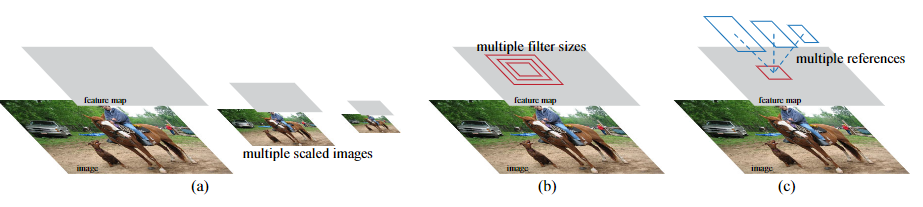Faster R-CNN is a single, unified network for object detection. The RPN module serves as the attention of this unified network.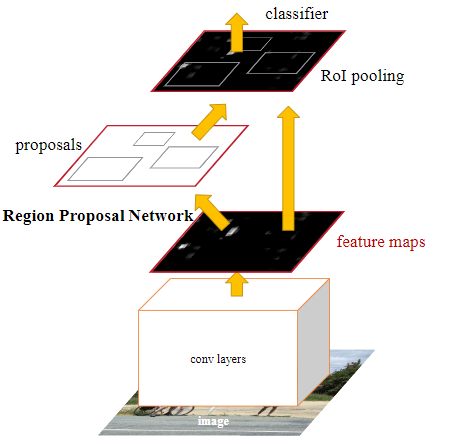RPN shares full-image convolutional features with the detection network, thus enabling nearly cost-free region proposals by attention mechanisms.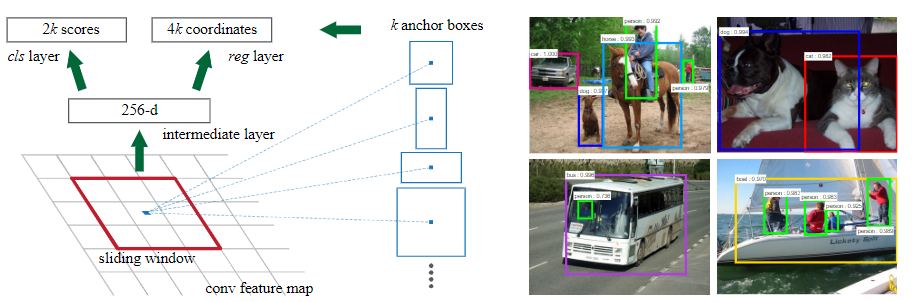Codes:
Proposal layer in RPN:

class _ProposalLayer(nn.Module):
"""
Outputs object detection proposals by applying estimated bounding-box
transformations to a set of regular boxes (called "anchors").
"""
def forward(self, input):

# Algorithm:
#
# for each (H, W) location i
#   generate A anchor boxes centered on cell i
#   apply predicted bbox deltas at cell i to each of the A anchors
# clip predicted boxes to image
# remove predicted boxes with either height or width < threshold
# sort all (proposal, score) pairs by score from highest to lowest
# take top pre_nms_topN proposals before NMS
# apply NMS with threshold 0.7 to remaining proposals
# take after_nms_topN proposals after NMS
# return the top proposals (-> RoIs top, scores top)

# the first set of _num_anchors channels are bg probs
# the second set are the fg probs
scores = input[:, self._num_anchors:, :, :]
bbox_deltas = input
im_info = input
cfg_key = input

pre_nms_topN  = cfg[cfg_key].RPN_PRE_NMS_TOP_N
post_nms_topN = cfg[cfg_key].RPN_POST_NMS_TOP_N
nms_thresh    = cfg[cfg_key].RPN_NMS_THRESH
min_size      = cfg[cfg_key].RPN_MIN_SIZE

batch_size = bbox_deltas.size(0)

feat_height, feat_width = scores.size(2), scores.size(3)
shift_x = np.arange(0, feat_width) * self._feat_stride
shift_y = np.arange(0, feat_height) * self._feat_stride
shift_x, shift_y = np.meshgrid(shift_x, shift_y)
shifts = torch.from_numpy(np.vstack((shift_x.ravel(), shift_y.ravel(),
shift_x.ravel(), shift_y.ravel())).transpose())
shifts = shifts.contiguous().type_as(scores).float()

A = self._num_anchors
K = shifts.size(0)

self._anchors = self._anchors.type_as(scores)
# anchors = self._anchors.view(1, A, 4) + shifts.view(1, K, 4).permute(1, 0, 2).contiguous()
anchors = self._anchors.view(1, A, 4) + shifts.view(K, 1, 4)
anchors = anchors.view(1, K * A, 4).expand(batch_size, K * A, 4)

# Transpose and reshape predicted bbox transformations to get them
# into the same order as the anchors:

bbox_deltas = bbox_deltas.permute(0, 2, 3, 1).contiguous()
bbox_deltas = bbox_deltas.view(batch_size, -1, 4)

# Same story for the scores:
scores = scores.permute(0, 2, 3, 1).contiguous()
scores = scores.view(batch_size, -1)

# Convert anchors into proposals via bbox transformations
proposals = bbox_transform_inv(anchors, bbox_deltas, batch_size)

# 2. clip predicted boxes to image
proposals = clip_boxes(proposals, im_info, batch_size)
# proposals = clip_boxes_batch(proposals, im_info, batch_size)

# assign the score to 0 if it's non keep.
# keep = self._filter_boxes(proposals, min_size * im_info[:, 2])

# trim keep index to make it euqal over batch
# keep_idx = torch.cat(tuple(keep_idx), 0)

# scores_keep = scores.view(-1)[keep_idx].view(batch_size, trim_size)
# proposals_keep = proposals.view(-1, 4)[keep_idx, :].contiguous().view(batch_size, trim_size, 4)

# _, order = torch.sort(scores_keep, 1, True)

scores_keep = scores
proposals_keep = proposals
_, order = torch.sort(scores_keep, 1, True)

output = scores.new(batch_size, post_nms_topN, 5).zero_()
for i in range(batch_size):
# # 3. remove predicted boxes with either height or width < threshold
# # (NOTE: convert min_size to input image scale stored in im_info)
proposals_single = proposals_keep[i]
scores_single = scores_keep[i]

# # 4. sort all (proposal, score) pairs by score from highest to lowest
# # 5. take top pre_nms_topN (e.g. 6000)
order_single = order[i]

if pre_nms_topN > 0 and pre_nms_topN < scores_keep.numel():
order_single = order_single[:pre_nms_topN]

proposals_single = proposals_single[order_single, :]
scores_single = scores_single[order_single].view(-1,1)

# 6. apply nms (e.g. threshold = 0.7)
# 7. take after_nms_topN (e.g. 300)
# 8. return the top proposals (-> RoIs top)

keep_idx_i = nms(torch.cat((proposals_single, scores_single), 1), nms_thresh, force_cpu=not cfg.USE_GPU_NMS)
keep_idx_i = keep_idx_i.long().view(-1)

if post_nms_topN > 0:
keep_idx_i = keep_idx_i[:post_nms_topN]
proposals_single = proposals_single[keep_idx_i, :]
scores_single = scores_single[keep_idx_i, :]

# padding 0 at the end.
num_proposal = proposals_single.size(0)
output[i,:,0] = i
output[i,:num_proposal,1:] = proposals_single

return output

Anchor target layer in RPN:
class _AnchorTargetLayer(nn.Module):
"""
Assign anchors to ground-truth targets. Produces anchor classification
labels and bounding-box regression targets.
"""
https://github.com/jwyang/faster-rcnn.pytorch/blob/master/lib/model/rpn/anchor_target_layer.py

Full RPN module:
class _RPN(nn.Module):
""" region proposal network """
def forward(self, base_feat, im_info, gt_boxes, num_boxes):
batch_size = base_feat.size(0)

# return feature map after convrelu layer
rpn_conv1 = F.relu(self.RPN_Conv(base_feat), inplace=True)
# get rpn classification score
rpn_cls_score = self.RPN_cls_score(rpn_conv1)

rpn_cls_score_reshape = self.reshape(rpn_cls_score, 2)
rpn_cls_prob_reshape = F.softmax(rpn_cls_score_reshape, 1)
rpn_cls_prob = self.reshape(rpn_cls_prob_reshape, self.nc_score_out)

# get rpn offsets to the anchor boxes
rpn_bbox_pred = self.RPN_bbox_pred(rpn_conv1)

# proposal layer
cfg_key = 'TRAIN' if self.training else 'TEST'

rois = self.RPN_proposal((rpn_cls_prob.data, rpn_bbox_pred.data,
im_info, cfg_key))

self.rpn_loss_cls = 0
self.rpn_loss_box = 0

# generating training labels and build the rpn loss
if self.training:
assert gt_boxes is not None

rpn_data = self.RPN_anchor_target((rpn_cls_score.data, gt_boxes, im_info, num_boxes))

# compute classification loss
rpn_cls_score = rpn_cls_score_reshape.permute(0, 2, 3, 1).contiguous().view(batch_size, -1, 2)
rpn_label = rpn_data.view(batch_size, -1)

rpn_keep = Variable(rpn_label.view(-1).ne(-1).nonzero().view(-1))
rpn_cls_score = torch.index_select(rpn_cls_score.view(-1,2), 0, rpn_keep)
rpn_label = torch.index_select(rpn_label.view(-1), 0, rpn_keep.data)
rpn_label = Variable(rpn_label.long())
self.rpn_loss_cls = F.cross_entropy(rpn_cls_score, rpn_label)
fg_cnt = torch.sum(rpn_label.data.ne(0))

rpn_bbox_targets, rpn_bbox_inside_weights, rpn_bbox_outside_weights = rpn_data[1:]

# compute bbox regression loss
rpn_bbox_inside_weights = Variable(rpn_bbox_inside_weights)
rpn_bbox_outside_weights = Variable(rpn_bbox_outside_weights)
rpn_bbox_targets = Variable(rpn_bbox_targets)

self.rpn_loss_box = _smooth_l1_loss(rpn_bbox_pred, rpn_bbox_targets, rpn_bbox_inside_weights,
rpn_bbox_outside_weights, sigma=3, dim=[1,2,3])

return rois, self.rpn_loss_cls, self.rpn_loss_box

Mask region-based convolutional network method (Mask R-CNN) extends Faster R-CNN by adding a branch for predicting an object mask in parallel with the existing branch for bounding box recognition.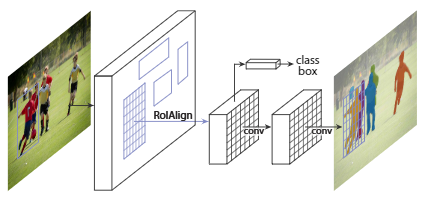Fast R-CNN has a class label and bounding-box offset output. Mask R-CNN further add a branch that output the object mask. But the additional mask output is distinct from the class and box outputs, requiring extraction of an object’s much more refined spatial layout.
Faster R-CNN heads were extended in two forms Codes: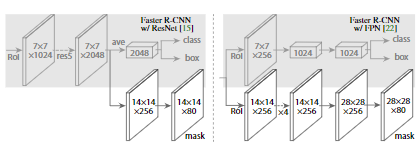# Create masks for detections
detection_boxes = KL.Lambda(lambda x: x[..., :4])(detections)
input_image_meta,
config.NUM_CLASSES,
train_bn=config.TRAIN_BN)

model = KM.Model([input_image, input_image_meta, input_anchors],
[detections, mrcnn_class, mrcnn_bbox,

# Run object detection
detections, _, _, mrcnn_mask, _, _, _ =\
self.keras_model.predict([molded_images, image_metas, anchors], verbose=0)

## FPN

Feature pyramids are a basic component in recognition systems for detecting objects at different scales. Feature pyramid network (FPN) is a top-down architecture with lateral connections developed for building high-level semantic feature maps at all scales.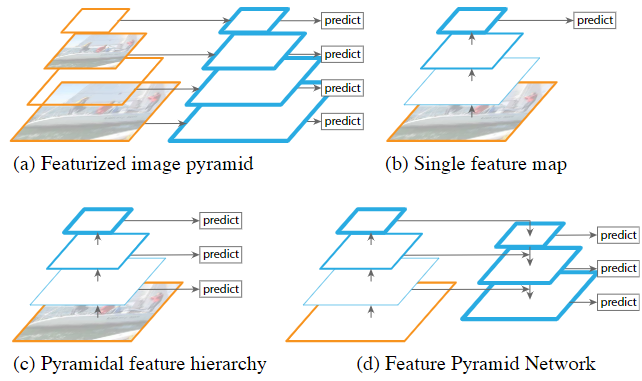Lateral connection and the top-down pathway, merged by addition.
The main modification of Fast R-CNN for FPN Codes:

class _FPN(nn.Module):
""" FPN """
'''Upsample and add two feature maps.
Args:
x: (Variable) top feature map to be upsampled.
y: (Variable) lateral feature map.
Returns:
Note in PyTorch, when input size is odd, the upsampled feature map
with F.upsample(..., scale_factor=2, mode='nearest')
maybe not equal to the lateral feature map size.
e.g.
original input size: [N,_,15,15] ->
conv2d feature map size: [N,_,8,8] ->
upsampled feature map size: [N,_,16,16]
So we choose bilinear upsample which supports arbitrary output sizes.
'''
_,_,H,W = y.size()
return F.upsample(x, size=(H,W), mode='bilinear') + y

def _PyramidRoI_Feat(self, feat_maps, rois, im_info):
''' roi pool on pyramid feature maps'''
# do roi pooling based on predicted rois
img_area = im_info * im_info
h = rois.data[:, 4] - rois.data[:, 2] + 1
w = rois.data[:, 3] - rois.data[:, 1] + 1
roi_level = torch.log(torch.sqrt(h * w) / 224.0)
roi_level = torch.round(roi_level + 4)
roi_level[roi_level < 2] = 2
roi_level[roi_level > 5] = 5
# roi_level.fill_(5)
if cfg.POOLING_MODE == 'crop':
# pdb.set_trace()
# pooled_feat_anchor = _crop_pool_layer(base_feat, rois.view(-1, 5))
# NOTE: need to add pyrmaid
grid_xy = _affine_grid_gen(rois, base_feat.size()[2:], self.grid_size)
grid_yx = torch.stack([grid_xy.data[:,:,:,1], grid_xy.data[:,:,:,0]], 3).contiguous()
roi_pool_feat = self.RCNN_roi_crop(base_feat, Variable(grid_yx).detach())
if cfg.CROP_RESIZE_WITH_MAX_POOL:
roi_pool_feat = F.max_pool2d(roi_pool_feat, 2, 2)

elif cfg.POOLING_MODE == 'align':
roi_pool_feats = []
box_to_levels = []
for i, l in enumerate(range(2, 6)):
if (roi_level == l).sum() == 0:
continue
idx_l = (roi_level == l).nonzero().squeeze()
box_to_levels.append(idx_l)
scale = feat_maps[i].size(2) / im_info
feat = self.RCNN_roi_align(feat_maps[i], rois[idx_l], scale)
roi_pool_feats.append(feat)
roi_pool_feat = torch.cat(roi_pool_feats, 0)
box_to_level = torch.cat(box_to_levels, 0)
idx_sorted, order = torch.sort(box_to_level)
roi_pool_feat = roi_pool_feat[order]

elif cfg.POOLING_MODE == 'pool':
roi_pool_feats = []
box_to_levels = []
for i, l in enumerate(range(2, 6)):
if (roi_level == l).sum() == 0:
continue
idx_l = (roi_level == l).nonzero().squeeze()
box_to_levels.append(idx_l)
scale = feat_maps[i].size(2) / im_info
feat = self.RCNN_roi_pool(feat_maps[i], rois[idx_l], scale)
roi_pool_feats.append(feat)
roi_pool_feat = torch.cat(roi_pool_feats, 0)
box_to_level = torch.cat(box_to_levels, 0)
idx_sorted, order = torch.sort(box_to_level)
roi_pool_feat = roi_pool_feat[order]

return roi_pool_feat

def forward(self, im_data, im_info, gt_boxes, num_boxes):
batch_size = im_data.size(0)

im_info = im_info.data
gt_boxes = gt_boxes.data
num_boxes = num_boxes.data

# feed image data to base model to obtain base feature map
# Bottom-up
c1 = self.RCNN_layer0(im_data)
c2 = self.RCNN_layer1(c1)
c3 = self.RCNN_layer2(c2)
c4 = self.RCNN_layer3(c3)
c5 = self.RCNN_layer4(c4)
# Top-down
p5 = self.RCNN_toplayer(c5)
p4 = self.RCNN_smooth1(p4)
p3 = self.RCNN_smooth2(p3)
p2 = self.RCNN_smooth3(p2)

p6 = self.maxpool2d(p5)

rpn_feature_maps = [p2, p3, p4, p5, p6]
mrcnn_feature_maps = [p2, p3, p4, p5]

rois, rpn_loss_cls, rpn_loss_bbox = self.RCNN_rpn(rpn_feature_maps, im_info, gt_boxes, num_boxes)

# if it is training phrase, then use ground trubut bboxes for refining
if self.training:
roi_data = self.RCNN_proposal_target(rois, gt_boxes, num_boxes)
rois, rois_label, gt_assign, rois_target, rois_inside_ws, rois_outside_ws = roi_data

## NOTE: additionally, normalize proposals to range [0, 1],
#        this is necessary so that the following roi pooling
#        is correct on different feature maps
# rois[:, :, 1::2] /= im_info
# rois[:, :, 2::2] /= im_info

rois = rois.view(-1, 5)
rois_label = rois_label.view(-1).long()
gt_assign = gt_assign.view(-1).long()
pos_id = rois_label.nonzero().squeeze()
gt_assign_pos = gt_assign[pos_id]
rois_label_pos = rois_label[pos_id]
rois_label_pos_ids = pos_id

rois_pos = Variable(rois[pos_id])
rois = Variable(rois)
rois_label = Variable(rois_label)

rois_target = Variable(rois_target.view(-1, rois_target.size(2)))
rois_inside_ws = Variable(rois_inside_ws.view(-1, rois_inside_ws.size(2)))
rois_outside_ws = Variable(rois_outside_ws.view(-1, rois_outside_ws.size(2)))
else:
## NOTE: additionally, normalize proposals to range [0, 1],
#        this is necessary so that the following roi pooling
#        is correct on different feature maps
# rois[:, :, 1::2] /= im_info
# rois[:, :, 2::2] /= im_info

rois_label = None
gt_assign = None
rois_target = None
rois_inside_ws = None
rois_outside_ws = None
rpn_loss_cls = 0
rpn_loss_bbox = 0
rois = rois.view(-1, 5)
pos_id = torch.arange(0, rois.size(0)).long().type_as(rois).long()
rois_label_pos_ids = pos_id
rois_pos = Variable(rois[pos_id])
rois = Variable(rois)

# pooling features based on rois, output 14x14 map
roi_pool_feat = self._PyramidRoI_Feat(mrcnn_feature_maps, rois, im_info)

## Shortcoming

Region proposal-based frameworks are composed of several correlated stages, including region proposal generation, feature extraction with CNN, classification, and bounding box regression, which are usually trained separately. Even in the recent end-to-end module Faster R-CNN, alternative training is still required to obtain shared convolution parameters between RPN and the detection network. As a result, the time spent handling different components becomes the bottleneck in the real-time application.

# Regression/Classification based framework

To be continued in Object detection and detection heads 2

# Reference

 Zhao, Z.Q., Zheng, P., Xu, S.T. and Wu, X., 2019. Object detection with deep learning: A review. IEEE transactions on neural networks and learning systems, 30(11), pp.3212-3232.
 Zou, Z., Shi, Z., Guo, Y. and Ye, J., 2019. Object detection in 20 years: A survey. arXiv preprint arXiv:1905.05055.
 He, K., Zhang, X., Ren, S. and Sun, J., 2015. Spatial pyramid pooling in deep convolutional networks for visual recognition. IEEE transactions on pattern analysis and machine intelligence, 37(9), pp.1904-1916.
 yueruchen/sppnet-pytorch
 Girshick, R., Donahue, J., Darrell, T. and Malik, J., 2014. Rich feature hierarchies for accurate object detection and semantic segmentation. In Proceedings of the IEEE conference on computer vision and pattern recognition (pp. 580-587).
 Girshick, R., 2015. Fast r-cnn. In Proceedings of the IEEE international conference on computer vision (pp. 1440-1448).
 Ren, S., He, K., Girshick, R. and Sun, J., 2015. Faster r-cnn: Towards real-time object detection with region proposal networks. Advances in neural information processing systems, 28.
 jwyang/faster-rcnn.pytorch
 He, K., Gkioxari, G., Dollár, P. and Girshick, R., 2017. Mask r-cnn. In Proceedings of the IEEE international conference on computer vision (pp. 2961-2969).